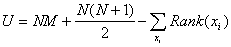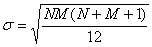# Mann-Whitney U-test

The Mann-Whitney U-test is a non-parametric method which is used as an alternative to the two-sample Student's t-test. Usually this test is used to compare medians of non-normal distributions X and Y (the t-test is not applicable because X and Y are not normal). The test works correctly under the following conditions:

• X and Y are continuous distributions (or discrete distributions well-approximating continuous distributions)
• X and Y have the same shape. The only possible difference is their position (i.e. the value of the median)
• the number of elements in each sample is not less than 5
• the samples are independent
• scale of measurement should be ordinal, interval or ratio (i.e. test could not be applied to nominal variables).

The MannWhitneyUTest subroutine returns three p-values:

• p-value for two-tailed test (null hypothesis - the medians are equal)
• p-value for left-tailed test (null hypothesis - the median of the first sample is greater than or equal to the median of the second sample)
• p-value for right-tailed test (null hypothesis - the median of the first sample is less than or equal to the median of the second sample)

Note #1
It's interesting to note that there are some views on what this test checks. The common interpretation is that the test checks the median equality. However, there are different points of view: for example, the U-test can be considered as checking if the probability of a random element from the first sample is greater than a random element from the second sample equals 0.5. One more (rare) interpretation is that this test checks if two distributions are equal. One part of a null hypothesis claims the equality of medians, but there is a second part as well which assumes the congruency of distribution shapes. Usually we assume that the first part is rejected by the test, but it denies both parts. However, this interpretation of U-test is very seldom used.

# Criterion

To make it simple, the U-test works as follows. Both samples (having sizes N and M) are combined into one array which is sorted in ascending order. We keep information about which sample the element had come from. After sorting, each element is replaced by its rank (its index in array, from 1 to N+M). Then the ranks of the first sample elements are summarized and the U-value is calculated:The mean of U equals 0.5·N·M. If U is close to this value, the medians of X and Y are close to each other. If we know distribution quantiles, we can get the significance level corresponding to the value of U.

# Distribution quantiles

Although U has discrete distribution if N and M are big it could be approximated by the normal distribution with a mean of 0.5·N·M and standard deviation:This approximation can be applied to most applications. For example, it can get good estimates for N, M > 10 and significance level α = 0.05 (most hypotheses are checked using these parameters). However, the distribution of U corresponds to the normal distribution only in the neighborhood of the central point (2-4 standard deviations depending on the values of N and M). Outside of this interval, the tails of the distribution go down more quickly than the tails of normal distribution. This means that if we use normal approximation to make a decision having α less than 0.001, we could accept a wrong null hypothesis. That's why all statistical programs don't use normal approximations for small M and N. Neither does ALGLIB. Furthermore, ALGLIB doesn't use normal approximation for bigger M and N either. Instead of that U is tabulated for small M and N (from 5 to 15), and for M, N > 15 asymptotic approximation is used. This method allows us to get p-values with satisfactory accuracy.

Note #2
The distribution of U could hardly be approximated. It is discrete, its integral function is not factorized neither in series nor in continued fractions. Therefore the code calculating the reciprocal of this distribution is very big: more than 100 Kb. I do not exactly know how this problem is solved by other authors (for example, in MatLab), but I think that it is better to have a bulky code than to have nothing.

Finally, some words about getting a distribution quantiles. The distribution of U has a peculiarity that if the null hypothesis is true, its integral function could be calculated by a finite number of steps using dynamic programming (it is true for a series of discrete distributions in non-parametric statistics). Thus, the main problem was to choose an appropriate approximation method. This problem was solved with the help of Chebyshev polynomials.

ALGLIB Project offers you two editions of ALGLIB:

ALGLIB Free Edition:delivered for freeoffers full set of numerical functionalityextensive algorithmic optimizationsno low level optimizationsnon-commercial license

ALGLIB Commercial Edition:flexible pricingoffers full set of numerical functionalityextensive algorithmic optimizationshigh performance (SMP, SIMD)commercial license with support plan

## ALGLIB 3.16.0 for C++C++ library.
Delivered with sources.
Monolithic design.
Extreme portability.
Editions:   FREE   COMMERCIAL

## ALGLIB 3.16.0 for C#C# library with native kernels.
Delivered with sources.
VB.NET and IronPython wrappers.
Extreme portability.
Editions:   FREE   COMMERCIAL

## ALGLIB 3.16.0 for DelphiDelphi wrapper around C core.
Delivered as precompiled binary.
Compatible with FreePascal.
Editions:   FREE   COMMERCIAL

## ALGLIB 3.16.0 for CPythonCPython wrapper around C core.
Delivered as precompiled binary.
Editions:   FREE   COMMERCIAL